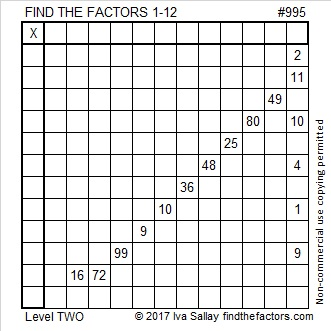# 995 and Level 2

If you know the only number that will divide evenly into 2, 11, 10, 4, 1, and 9, then you can easily solve this level 2 puzzle. There are even elementary aged kids that you know who can solve it. I’m sure that together you can fill in every cell in the entire puzzle. Don’t be afraid. Just do it.Print the puzzles or type the solution in this excel file: 12 factors 993-1001

Here’s some stuff that you probably didn’t know about the number 995:

995 has only two factor pairs, and they both use the exact same digits (1, 9, 9, 5):
1 × 995 =  199 × 5

It is the hypotenuse of a Pythagorean triple:
597-796-995 which is (3-4-5) times 199

It is palindrome 3E3 in BASE 16 (E is 14 base 10) because 3(16²) + 14(16) + 3(1) = 995

• 995 is a composite number.
• Prime factorization: 995 = 5 × 199
• The exponents in the prime factorization are 1 and 1. Adding one to each and multiplying we get (1 + 1)(1 + 1) = 2 × 2 = 4. Therefore 995 has exactly 4 factors.
• Factors of 995: 1, 5, 199, 995
• Factor pairs: 995 = 1 × 995 or 5 × 199
• 995 has no square factors that allow its square root to be simplified. √995 ≈ 31.54362This site uses Akismet to reduce spam. Learn how your comment data is processed.